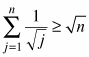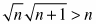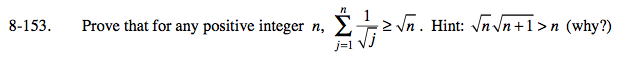### Home > PC > Chapter 8 > Lesson 8.2.6 > Problem8-153

8-153.

Prove that for any positive integer n,. Hint:(why?) Homework Help ✎$\frac{1}{\sqrt{1}}\geq \sqrt{1}$

$\text{Assume }\sum_{j=1}^{k}\frac{1}{\sqrt{j}}\geq \sqrt{k}\text{ for some integer }k.$

$\sum_{j=1}^{k+1}\frac{1}{\sqrt{j}}=$

$\sum_{j=1}^{k}\frac{1}{\sqrt{j}}+\frac{1}{\sqrt{k+1}}\geq$

$\sqrt{k}+\frac{1}{\sqrt{k+1}}=$

$\frac{\sqrt{k}\sqrt{k+1}+1}{\sqrt{k+1}}\geq$

$\frac{k+1}{\sqrt{k+1}}= {\sqrt{k+1}}$

So the induction step is true.

Hence, by mathematical induction we have proven that

$\sum_{j=1}^{n}\frac{1}{\sqrt{j}}\geq \sqrt{x}n$

for any positive integer n.